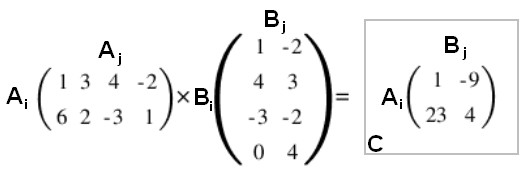# Linear Algebra - Row vector (One-row matrix)

A vector is interpreted as a one-column matrix (a column vector)

To become a one-row matrices, use transpose to turn a column vector into a row vector.

$$\begin{array}{c} \text{Suppose } {\bf b} = [1, 2, 3] \\ [1,2,3] * A = [1 2 3] * \begin{bmatrix} & & \\ & \large{A} & \\ & & & \end{bmatrix} = {\bf b}^T A \end{array}$$

Discover MoreLinear Algebra - Column Vector (One-column matrix)

A vector can be (seen|interpreted) as a one-column matrix. To get a one-row matrix, use . Multiplying a matrix A by a one-column matrix B: By matrix-vector definition of matrix-matrix multiplication,...Linear Algebra - Matrix

The Traditional notion of a matrix is: a two-dimensional array a rectangular table of known or unknown numbers One simple role for a matrix: packing together a bunch of columns or rows Matrix...Linear Algebra - Matrix Matrix (Multiplication)

Matrix Matrix (Multiplication) definition . Two matrices may be multiplied when they are conformable: ie the number of columns in the first matrix is equal to the number of rows in the second matrix....